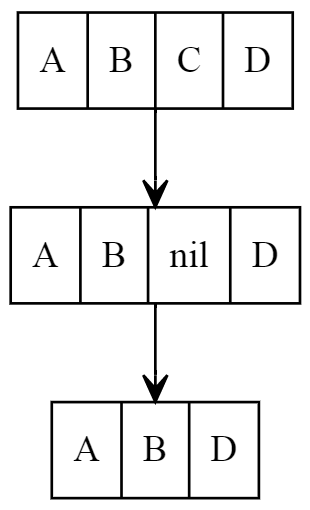# Practical Linked List in Ruby

This is the 3rd entry in the “Practical Computer Science in Ruby” series! Today we are going to talk about linked list.

Like the name says, a linked list is a way to store data in a list format (thanks, Captain Obvious!).

The “linked” part comes from the fact that data is stored in a node & these nodes are linked to each other in a sequential manner.How is this different from an array?

A linked list has different performance characteristics than an array. That’s one reason why you may want to pick one over the other.

This means that a linked list can be more efficient for certain tasks than an array.

In a linked list there is no indexing, no random access, meaning that you can’t reach out for an item in the middle of the list.

You have to start at the “head” of the list & follow the links until you find the node you want or until the end of the list.

Removing (or adding) an item from the middle of a linked list is a lot faster.

All you have to do is change the “next” pointer for one node.

But if you want to delete an element from the middle of an array you will leave a gap & to close that gap you have to move all the elements on the right of the deleted element.Not very efficient if you have to do this often!

If we want to insert in the middle of an array we have to move all the array elements to create an empty space.

Let’s see a code example:

```a = [1,2,3,4,5,6,7,8]

def insert(arr, item, pos)
tmp      = arr[pos]
arr[pos] = item

arr.replace(arr[0..pos] + [tmp] + arr[pos+1..-1])
end

insert(a, 99, 3)
p a
```

Note: There is also a built-in Array#insert method, but I wanted to show you how this method works.

What is the performance impact of inserting an item in the middle of a list?

Here’s a benchmark:

```Comparison:
Array:       18090.3 i/s - 100.35x  slower
```

Big difference here, but it also depends a lot on the size of the `LinkedList` since we have to search for the node.

Another way to compare two data structures is to look at a time complexity table (this assumes you don’t have to search a node for insertion & deletion):

Data Structure Access Search Insertion Deletion
Array O(1) O(n) O(n) O(n)
Linked List O(n) O(n) O(1) O(1)

Looking for real-world applications?

Here’s a pull request by Aaron Patterson where he speeds up Ruby Gems by using a linked list:

https://github.com/rubygems/rubygems/pull/1188

Ruby doesn’t include a `LinkedList` class so we need to create our own.

We want the following operations to be available:

• append (to the end of the list)
• append_after
• delete
• find

Here’s one possible implementation:

```class LinkedList
def initialize
end

def append(value)
find_tail.next = Node.new(value)
else
end
end

def find_tail

return node if !node.next
return node if !node.next while (node = node.next)
end

def append_after(target, value)
node           = find(target)

return unless node

old_next       = node.next
node.next      = Node.new(value)
node.next.next = old_next
end

def find(value)

return false if !node.next
return node  if node.value == value

while (node = node.next)
return node if node.value == value
end
end

def delete(value)
return
end

node      = find_before(value)
node.next = node.next.next
end

def find_before(value)

return false if !node.next
return node  if node.next.value == value

while (node = node.next)
return node if node.next && node.next.value == value
end
end

def print
puts node

while (node = node.next)
puts node
end
end
end
```

This particular implementation doesn’t keep track of the tail, it finds the tail when appending a new item. This makes the append operation linear time (`O(n)`). In the video below I show you another implementation where I append the elements at the front.

And here is our node class:

```class Node
attr_accessor :next

def initialize(value)
@value = value
@next  = nil
end

def to_s
"Node with value: #{@value}"
end
end
```

We can use it like this:

```list = LinkedList.new

list.append(10)
list.append(20)
list.append(30)

list.append_after(10, 15)
list.append_after(20, 25)

list.print
```

This is a basic “Singly-Linked List” implementation.

You also have other types of linked list:

In the doubly-linked list every node has two pointers: one to the next node & another to the previous node.

This allows for more flexibility when searching the list, but also requires extra work when making changes to the list.

A circular linked list is the same than a doubly-linked list, but the last node is connected to the head node.

## Summary

You have learned about linked lists, a data structure which you could consider if you are doing a lot of adding & removing of elements in the middle of an array.

It could also come up in a coding interview, so it’s worth learning even if it’s just for that.

Don’t forget to share this post using the buttons below so more people can benefit from this knowledge 🙂

Oliver Kiessler says 3 years ago

Useful article, thanks for sharing!

Jesus Castello says 3 years ago